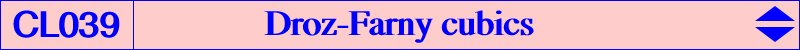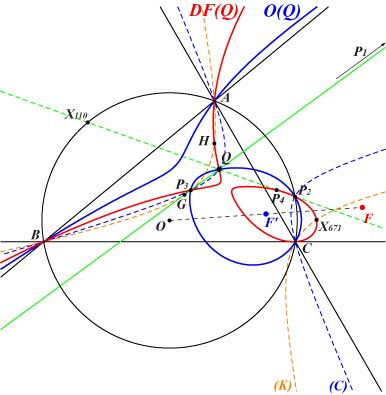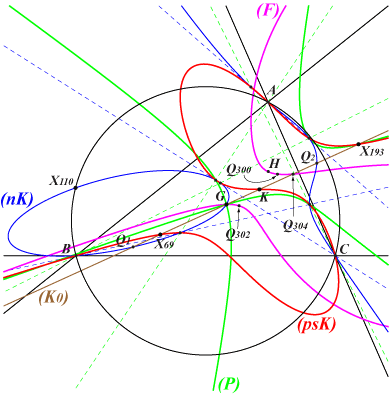Consider two perpendicular lines L and L' passing through H, intersecting the sidelines of ABC at U, V, W and U', V', W' respectively. The midpoints A', B', C' of UU', VV', WW' are always collinear on a line ∆. We say that L, L' always verify the Droz-Farny property. When L and L' rotate about H the envelope of ∆ is the MacBeath conic i.e. the inconic with foci O, H and center X(5). ∆ meets L, L' at two points that lie on the homothetic of K406 under h(H, 1/2). Now, if L and L' are secant at P different of H, there is only one pair of such lines verifying the DF-property which we call DF-lines of P. These DF-lines are actually the parallels at P to the asymptotes of the rectangular circum-hyperbola that contains P. In this case, the line L_P through these midpoints is called the DF-axis of P and the trilinear pole P' of L_P is said to be the DF-pole of P. More information about DF-lines in the FG papers "A Synthetic Proof of the Droz-Farny Line Theorem" by J.-L. Ayme and "A Projective Generalization of the Droz-Farny Line Theorem" by Jean-Pierre Erhmann and Floor van Lamoen. See also the related quintic Q044. With P = p : q : r, the DF-pole is : P' = 2 SA p (p + q + r) - (a^2 q r + b^2 r p + c^2 p q) : : . This shows that the DF-pole of any point at infinity is the centroid G and the DF-pole of any point on the circumcircle lies on the circum-conic with perspector O. P' lies on the line through G, the barycentric quotient P÷H and the orthocorrespondent P⊥ of P which is the point with barycentric coordinates : P⊥ = SA p (p + q + r) - (a^2 q r + b^2 r p + c^2 p q) : : . P' is in fact the harmonic conjugate of G with respect to P÷H and P⊥. The mapping P –> P' is quadratic. It has three singular points (H and the circular points at infinity) and four fixed points (A, B, C and X(671), the antipode of X(99) on the Steiner ellipse). Let Q = u : v : w be a fixed point. The locus of P such that P, P', Q are collinear is a circular circum-cubic passing through H and X(671). We say that this cubic is a DF-cubic which we denote by DF(Q). All DF-cubics form a net of circular cubics. *** Geometric properties DF(Q) contains Q and the infinite point P1 of the line GQ (when Q = G, the cubic decomposes into the line at infinity and the Kiepert hyperbola). DF(Q) meets the circumcircle again at P2 on the line through Q and X(110) (when Q = X(110), the cubic decomposes into the line H-X(671) and the circumcircle). DF(Q) meets the Kiepert hyperbola (K) at A, B, C, H, X(671) and P3 on the line through Q and G. The third point P4 of DF(Q) on the line H, X(671) also lies on the line through Q and X(110). DF(Q) meets the Steiner ellipse at A, B, C, X(671) and two other points S1, S2 which lie on the trilinear polar of the isotomic conjugate of the complement of Q. These points are real if and only if Q lies outside the Steiner ellipse. They coincide if and only if Q lies on the Steiner ellipse.Let O(Q) be the orthopivotal cubic with orthopivot Q ≠ G, Q ≠ X(110). O(Q) passes through nine identified points of DF(Q) namely A, B, C, Q, P1, P2, P3 and the circular points at infinity. It follows that DF(Q) and O(Q) generate a pencil of cubics which contains two decomposed cubics : • the union of the circumcircle and the line GQ, • the union of the line at infinity and the circum-conic (C) passing through Q, P2, P3. Let F, F' be the singular foci of DF(Q) and O(Q) respectively. The construction of F' is given in the page orthopivotal cubic and F is the reflection of O about F'. For instance, when Q = X3, the cubics are DF(X3) = K854, O(X3) = K001 with foci X(399), X(110) respectively.The following table shows a selection of pairs DF(Q), O(Q) with corresponding foci F, F'.Q X69 X4 X193 X6 X23 X100 Q300 X3448 Q302 X15 X16 Q304 X511 X20 X3 DF(Q) K008 K025 K209 K273 K298 K299 K300 K301 K302 K303a K303b K304 K305 K809 K854 O(Q) K442 K059 K018 K262a K262b K263 K313 K001 F X10748 X265 X5512 X11258 X6 X14661 X671 X4 X23 X5615 X5611 X14662 X381 X113 X399 F' X126 X125 X6719 X111 X182 X1083 ? X5 X7575 X16 X15 ? X2 X5972 X110Notes : Q300 = X(4)X(94) /\ X(6)X(23), SEARCH = -0.715622153886245, now X(11002) in ETC (2016-11-22) Q302 = X(2)X(98) /\ X(6)X(23), SEARCH = 11.9769821093760, now X(11003) in ETC (2016-11-22) Q304 = X(2)X(6) /\ X(4)X(195), SEARCH = -9.52782777900564, now X(11004) in ETC (2016-11-22)*** Special DF-cubics 1. DF(Q) is a K0 cubic (without term in xyz) if and only if Q lies on the line GK. In this case, the singular focus lies on the line X(114)-X(381) and all the cubics form a pencil of cubics through A, B, C, G, H, X(524), X(671) and the circular points at infinity. See the orange cells in the table below. 2. DF(Q) is a focal if and only if Q lies on a cubic (F) passing through G, H, X(23), X(3448) and several other points described in the notes below the table. In this case, the singular focus lies on the cubic K300 which is also a DF-cubic. DF(G) is decomposed. DF(H) = K025 = Ehrmann strophoid. DF(X23) = K298. 3. DF(Q) is a psK if and only if Q lies on a central cubic denoted (psK) with center X(6), passing through X(69), X(193). 4. DF(Q) is a nK if and only if Q lies on a cubic denoted (nK) passing through X(2), X(110) giving two decomposed cubics. The former is the union of the line at infinity and the Kiepert hyperbola, the latter is the union of the line X(4)X(671) and the circumcircle.In particular, DF(Q) is a pK if and only if either : • Q = K then DF(Q) = K273 = pK(X111, X671), • Q = X(69) then DF(Q) = K008 = pK(X2, X316), the Droussent cubic, • Q = X(193) then DF(Q) = K209 = pK(X468, X4), and a nK0 if and only if either : • Q = G, decomposed cubic, • Q is one of the points Q1, Q2 with very complicated coordinates.   5. DF(Q) meets the sidelines of ABC at the vertices of a pedal triangle if and only if Q lies on a nodal cubic (P) with node G, passing through X(193) corresponding to the cubic K209.6. When Q lies at infinity, DF(Q) has singular focus X(381), the midpoint of GH, and meets the Steiner ellipse again at S1, S2 which are now antipodes on the ellipse. These cubics form a pencil which contains only one focal cubic. See K305 = DF(X511) for example. 7. DF(Q) is a singular cubic if and only if Q lies on a 12th degree curve which contains G, H, X(110) and X(671). DF(H) = K025 is the Ehrmann strophoid and DF(X671) has node X(671). *** The following table presents a selection of DF-cubics with generally at least six ETC centers on the curve. F is the singular focus.Q Centers on the cubic cubic remark F X(1) X(1), X(4), X(10), X(519), X(671), X(759), X(1320) X(3) X(3), X(4), X(30), X(74), X(671) K854 X(399) X(4) X(4), X(30), X(265), X(316), X(671), X(1263), X(1300) K025 strophoid X(265) X(6) X(2), X(4), X(6), X(23), X(111), X(524), X(671), X(895) K273 pK X(11258) X(8) X(4), X(8), X(10), X(80), X(519), X(671) X(9) X(4), X(9), X(226), X(527), X(671), X(1156) X(15) X(4), X(14), X(15), X(531), X(671), X(1337), X(2378) K303a X(5615) X(16) X(4), X(13), X(16), X(530), X(671), X(1338), X(2379) K303b X(5611) X(20) X(4), X(20), X(30), X(671), X(1294) K809 X(113) X(23) X(4), X(6), X(23), X(30), X(671), X(842) K298 focal X(6) X(32) X(4), X(32), X(83), X(671), X(729), X(754) X(39) X(4), X(39), X(76), X(538), X(671), X(755) X(54) X(4), X(54), X(96), X(539), X(671), X(1141) X(61) X(4), X(18), X(61), X(533), X(671), X(2380) X(62) X(4), X(17), X(62), X(532), X(671), X(2381) X(69) X(2), X(4), X(67), X(69), X(316), X(524), X(671), X(858), X(2373) K008 pK X(10748) X(76) X(4), X(76), X(316), X(538), X(671), X(2367) X(81) X(2), X(4), X(81), X(105), X(524), X(671), X(1325) X(86) X(2), X(4), X(86), X(524), X(671), X(675) X(100) X(4), X(100), X(190), X(528), X(664), X(671), X(1156), X(1320) K299 X(14661) X(146) X(4), X(146), X(265), X(541), X(671), X(1294) X(193) X(2), X(4), X(126), X(193), X(468), X(524), X(671), X(2374) K209 pK X(5512) X(251) X(4), X(83), X(251), X(671), X(733), X(754) X(274) X(4), X(76), X(274), X(538), X(671), X(767) X(315) X(4), X(83), X(315), X(316), X(671), X(754) X(323) X(2), X(3), X(4), X(323), X(524), X(671), X(842) X(333) X(2), X(4), X(333), X(524), X(671), X(1311) X(352) X(2), X(4), X(352), X(524), X(671), X(843) X(385) X(2), X(4), X(385), X(524), X(671), X(1316) X(392) X(4), X(8), X(104), X(392), X(517), X(671) X(394) X(2), X(4), X(394), X(524), X(671), X(1297), X(2071) X(511) X(4), X(262), X(316), X(511), X(671), X(842) K305 X(381) X(568) X(4), X(54), X(265), X(568), X(671), X(1154) X(621) X(4), X(14), X(316), X(531), X(621), X(671) X(622) X(4), X(13), X(316), X(530), X(622), X(671) X(633) X(4), X(18), X(316), X(533), X(633), X(671) X(634) X(4), X(17), X(316), X(532), X(634), X(671) X(1352) X(4), X(98), X(316), X(542), X(671), X(1352) X(3448) X(4), X(67), X(98), X(115), X(265), X(542), X(671) K301 focal X(4) Q300 X(4), X(6), X(23), X(262), X(265), X(381), X(511), X(576), X(671) K300 focal X(671) Q302 X(3), X(4), X(6), X(23), X(98), X(542), X(671), X(2080) K302 focal X(23) Q304 X(2), X(4), X(15), X(16), X(524), X(576), X(671), X(1263) K304 X(14662)